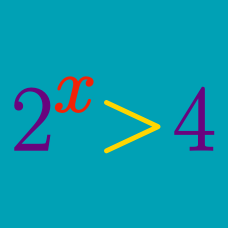Algebra

# Exponential Inequalities - Problem Solving

How many positive integers $x<1000$ satisfy the inequality $x^{3x+1} > x^{x+139}$?

Which of the following satisfies the inequality $3^y \geq 4^x - 2 ?$

How many integers $x$ satisfy the inequality $\frac{2 ^5}{5 ^5} < \left( \frac{2}{5} \right)^{x^2} < \frac{125}{8} \cdot \left( \frac{16}{625} \right)^x ?$

What is the minimum value of $x$ that satisfies the inequality $2^{2x} - 127 \cdot 2^x -128 \geq 0$?

If $y=f(x)$ is the equation of the line which has a slope of $-\frac{1}{5}$ and passes through the point $(0,5),$ what is the solution to the inequality $0 \leq f(5^x) \leq 10 ?$

×